Grid List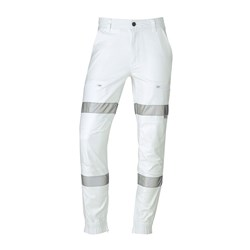\$94.95
Increase value Decrease value
\$99.95
Increase value Decrease value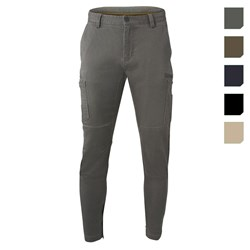E1191___ Grid Work Pant
Was: \$89.95 Now: \$77.95
Increase value Decrease value
\$69.95
Increase value Decrease value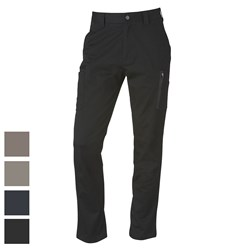\$89.95
Increase value Decrease value
\$89.95
Increase value Decrease value
\$59.95
Increase value Decrease value
\$40.00
Increase value Decrease value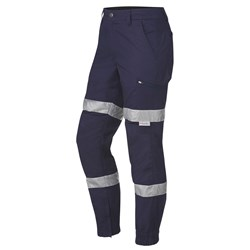Was: \$94.95 Now: \$82.95
Increase value Decrease value
\$79.95
Increase value Decrease value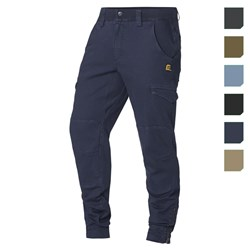Was: \$89.95 Now: \$77.95
Increase value Decrease value
\$59.95
Increase value Decrease value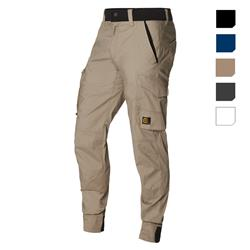Was: \$84.95 Now: \$72.95
Increase value Decrease value
\$74.95
Increase value Decrease value
\$89.95
Increase value Decrease value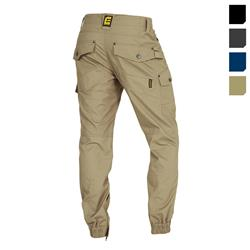Was: \$84.95 Now: \$72.95
Increase value Decrease value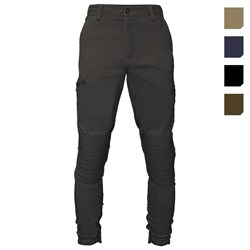Was: \$89.95 Now: \$77.95
Increase value Decrease value
\$69.95
Increase value Decrease value
\$84.95
Increase value Decrease value
EB1192__ Motion Pant
\$99.95
Increase value Decrease value
\$64.95
Increase value Decrease value
\$69.95
Increase value Decrease value
\$79.95
Increase value Decrease value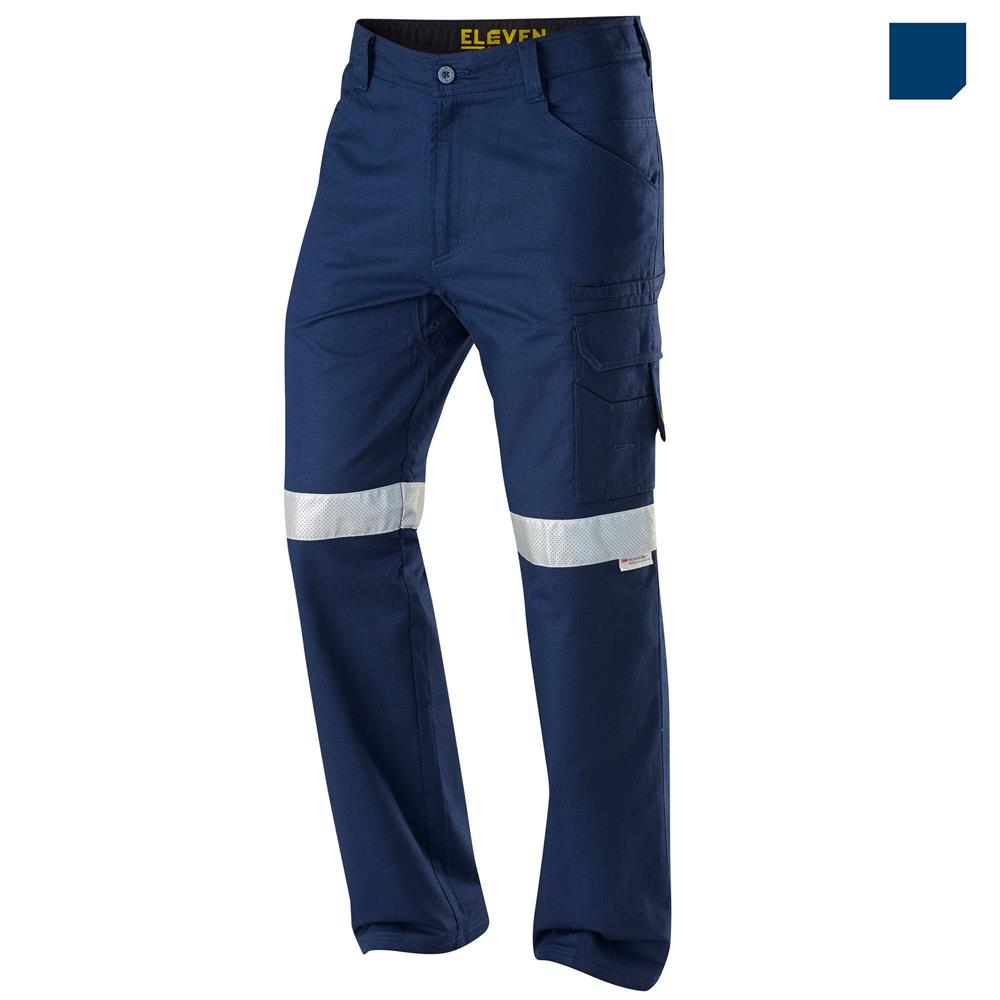Was: \$74.95 Now: \$64.95
Increase value Decrease value
Grid List